• c++函数模板参数推断
千次阅读
2020-10-28 14:17:26

c++模板类型推断分为类模板类型推断和函数模板类型推断,这里主要讨论的是c++函数模板推断.

函数模板类型推断情况分为三种:

##### 情况1：
template<typename T>
void func(T t)
{
//....
}


此种情况最为简单，对于传递给函数模板的实参val，假设其类型为ParaType，若ParaType具有引用属性，则去掉引用属性，若具有const属性也去掉，最终得到的类型即为T的类型：

int i = 999;
func(i);		//实参i既无引用属性，也无const属性，T类型为int

const int ci = 250;
func(ci);		//实参ci有const属性，去掉，T类型为int

const int &cref = 520;
func(cref);		//去掉实参cref引用属性和const属性，T类型为int

##### 情况2：
template<typename T>
void func(T &t)
{
//...
}


这种情况下，对于传递给函数模板的实参val，类型为ParaType，若ParaType具有引用属性，则去掉引用属性,若具有const属性则保留。

int i = 999;
func(i);		//实参i既无引用属性，也无const属性，T类型为int

const int ci = 250;
func(ci);		//实参ci有const属性，T类型为const int

const int &cref = 520;
func(cref);		//去掉实参cref引用属性，T类型为const int

const int &&crref = 6666;
func(crref);		//去掉实参crref引用属性，T类型为const int

##### 情况3：
template<typename T>
void func(T &&t)
{
//...
}


这种情况即为所谓的万能引用，实参类型可以为任意类型(左值、右值、左值引用、右值引用，虽然将模板函数声明为const T&也可以接收任意类型，但是存在不能修改实参的限制)。

对于传递给此函数模板的实参：

1. 当实参类型为左值 ** (注意左值、左值引用、右值引用均为左值) ** 时，T的类型会被推断为type&;当实参类型为右值时，T的类型会被推断为type。那type什么是类型呢？type即上述情况二中推导规则。
###### 举例：
std::string s("fuck");
func(s);		//s为左值，type为std::stirng ， T被推断为std::string &

const std::string &lref = s;
func(lref);		//lref为左值，type为const std::string T被推导为const std::string &

const std::string &&rref = std::string("fuck c++");
func(rref);		//同上

1. 当实参类型为右值时，T的类型被推断为实参相应类型。
###### 举例:
func(std::string("hello c++");		//T被推断为std::string

std::string s("give up c++");
func(std::move(s));		//T被推断为std::string

func(static_cast<std::string &&>(s));		//T被推断为 std::string


到此为止T的类型全部已知，直接将推断类型带入到函数模板即可得到实例化函数，例如：

//情况一
func(int t)		//T为int
{
//...
}

//情况二
func(const int &t)		//T为const int
{
//...
}

//情况三，实参为左值时
func(const std::string& &&t)		//T为const std::string &
{
//...
}

//情况三，实参为右值时
func(std::string &&t)		/T为std::string
{
//...
}


在情况三中，当实参为左值时其实例化结果有点奇怪，编译器并不允许程序员直接这样写，通过引用折叠，最终版本为：

//可以看到，当实参为左值类型(左值、左值引用、右值引用)时，最终t都会绑定到一个左值对象或右值对象上
func(const std::string &t)
{
//....
}

更多相关内容
• 其中，template为关键字，表示定义一个模板（可以是函数模板或类模板），尖括号表示模板参数，模板类型参数使用关键字class或typename开始，其后是一个用户定义的合法的标识符。 如果用户在调用函数模板时显式标识...
• 本文针对C++函数模板与类模板进行了较为详尽的实例解析，有助于帮助读者加深对C++函数模板与类模板的理解。具体内容如下： 泛型编程（Generic Programming）是一种编程范式，通过将类型参数化来实现在同一份代码上...
• 练习 C++函数模板、类模板的创建和使用方法。 (1) 理解模板的作用。 (2) 学习函数模板及其声明方法，掌握模板函数及其生成方法。 (3) 学习函数模板的两种不同的实例化方法。 (4) 学习类模板的声明与使用方法。
• 目录前言C++函数模板的使用函数模板语法1.模板说明2.函数定义3.函数模板调用模板函数函数模板和函数重载 前言 C++提供了模板(template)编程的概念。所谓模板，实际上是建立一个通用函数或类，其类内部的类型和函数的...

# 前言

C++提供了模板(template)编程的概念。所谓模板，实际上是建立一个通用函数或类，其类内部的类型和函数的形参类型不具体指定，用一个虚拟的类型来代表。这种通用的方式称为模板。模板是泛型编程的基础,泛型编程即以一种独立于任何特定类型的方式编写代码。

# C++函数模板的使用

为什么要有函数模板

例如，在一个项目中，有个项目需求是能够实现多个函数用来返回两个数的最大值，要求能支持char类型、int类型、double类型变量。然后呢，根据这个需求，我们写了以下这个代码。

#include <iostream>

using namespace std;

//比较int 类型
int Max(int a, int b)
{
return a > b ? a : b;
}

//比较char 类型
char Max(char a, char b)
{
return a > b ? a : b;
}

//比较float 类型
float Max(float a, float b)
{
return a > b ? a : b;
}

int main(void)
{

int  n = 1;
int	 m = 2;
cout << "max(1, 2) = " << Max(n, m) << endl;

float a = 2.0;
float b = 3.0;
cout << "max(2.0, 3.0) = " << Max(a, b) << endl;

char i = 'a';
char j = 'b';
cout << "max('a', 'b') = " << Max(i, j) << endl;

return 0;
}


执行得到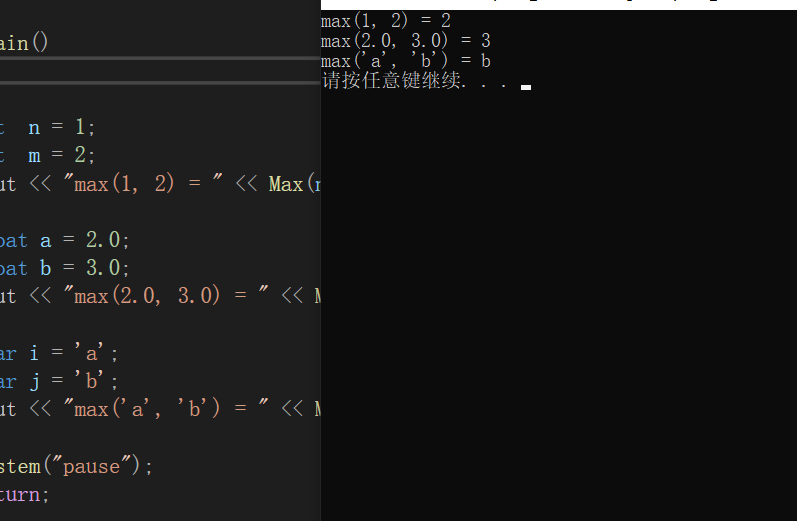我们看到，如果我们需要比较多个类型时，需要多个函数，而实际上我们只需要定义一个 “函数” 就能解决。

#include <iostream>

using namespace std;

//template 关键字告诉C++编译器 要开始泛型编程了
//T - 参数化数据类型
template <typename T>
T Max(T a, T b) {
return a > b ? a : b;
}

int main(void)
{

int  n = 1;
int	 m = 2;
cout << "max(1, 2) = " << Max(n, m) << endl;

float a = 2.0;
float b = 3.0;
cout << "max(2.0, 3.0) = " << Max(a, b) << endl;

char i = 'a';
char j = 'b';
cout << "max('a', 'b') = " << Max(i, j) << endl;

return 0;
}


得到的结果是一样的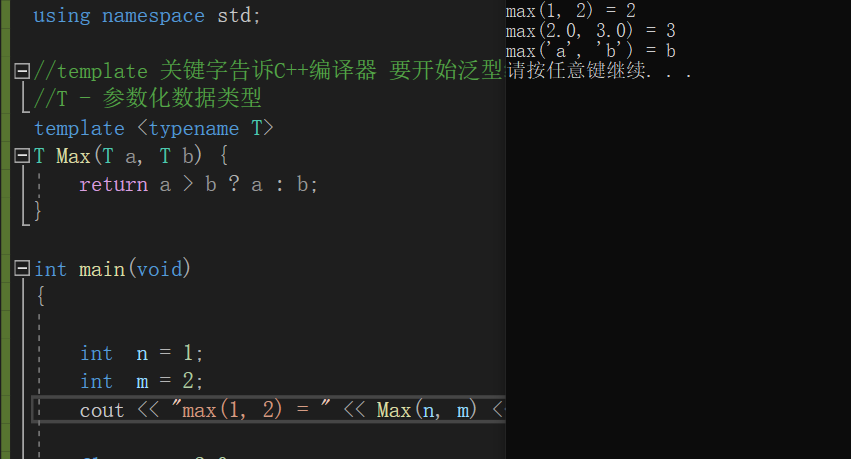我们在定义一个函数模板之后，，没有指定类型，编译器会实现参数类型的自动推导。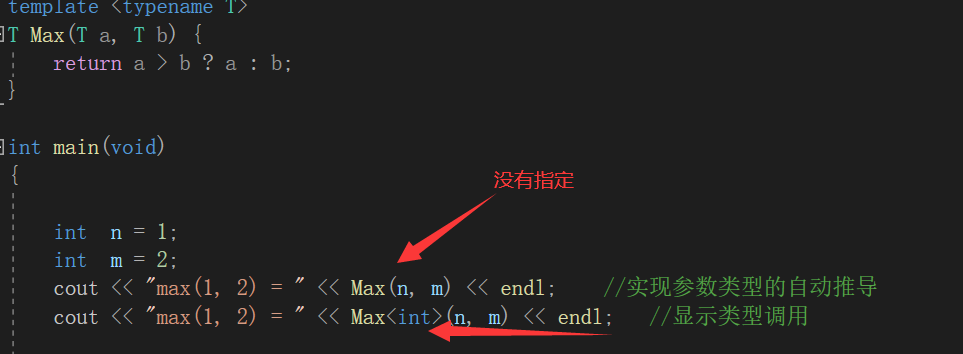# 函数模板语法

所谓函数模板，实际上是建立一个通用函数，其函数类型和形参类型不具体指定，用一个虚拟的类型来代表。这个通用函数就称为函数模板。

凡是函数体相同的函数都可以用这个模板来代替，不必定义多个函数，只需在模板中定义一次即可。在调用函数时系统会根据实参的类型来取代模板中的虚拟类型，从而实现了不同函数的功能。

函数模板定义形式
由以下三部分组成： 模板说明 + 函数定义 + 函数模板调用

template < 类型形式参数表 >
类型 函数名 (形式参数表)
{
//语句序列
}

我们也可以定义多个类型

template <typename T, typename T2>


但是我们定义了一定要用，否则会报错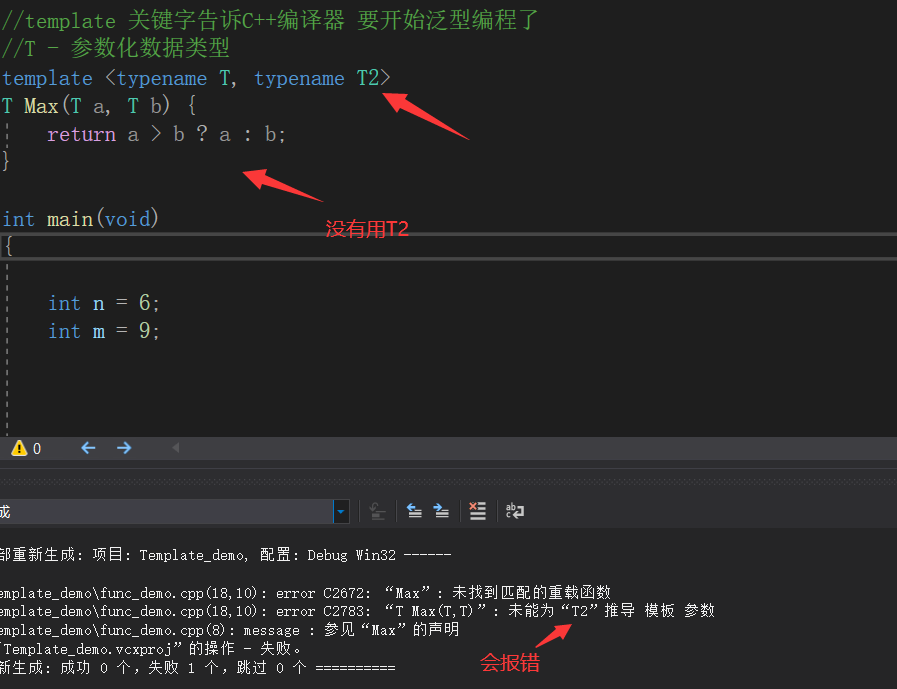将代码改成

#include <iostream>

using namespace std;

//template 关键字告诉C++编译器 要开始泛型编程了
//T - 参数化数据类型
template <typename T, typename T2>
T Max(T a, T2 b) {
return a > b ? a : b;
}

int main(void){

int n = 6;
int m = 9;

cout << Max(n, m) << endl;

system("pause");
return 0;
}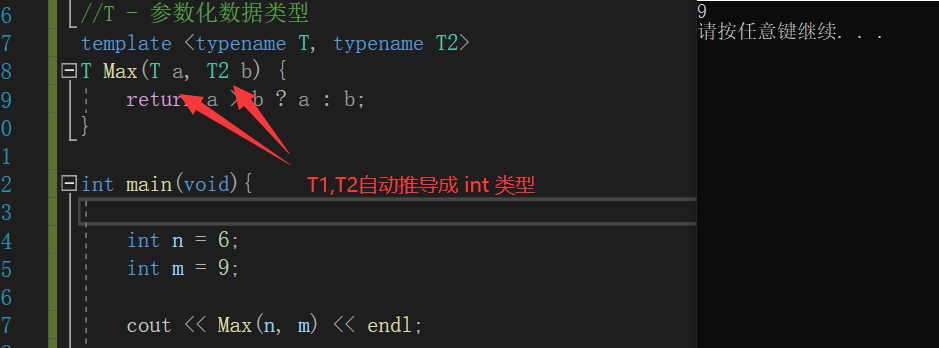## 1.模板说明

template < 类型形式参数表 >
类型形式参数的形式：
typename T1 , typename T2 , …… , typename Tn
或 class T1 , class T2 , …… , class Tn
注意： typenameclass 的效果是一样的，不过建议呢使用typename，为了防止用class有歧义）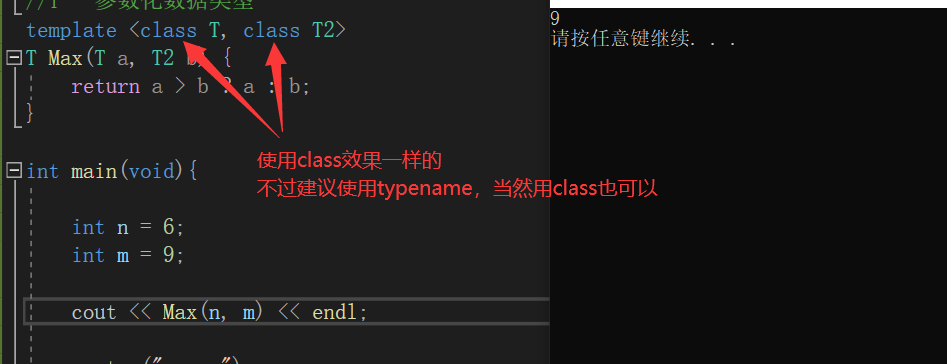## 2.函数定义

类型 函数名 (形式参数表)
{
}
注意： 模板说明的类属参数必须在函数定义中出现一次,函数参数表中可以使用类属类型参数，也可以使用一般类型参数

## 3.函数模板调用

Max<int,int>(a, b); //显式类型调用
Max(a, b); //自动数据类型推导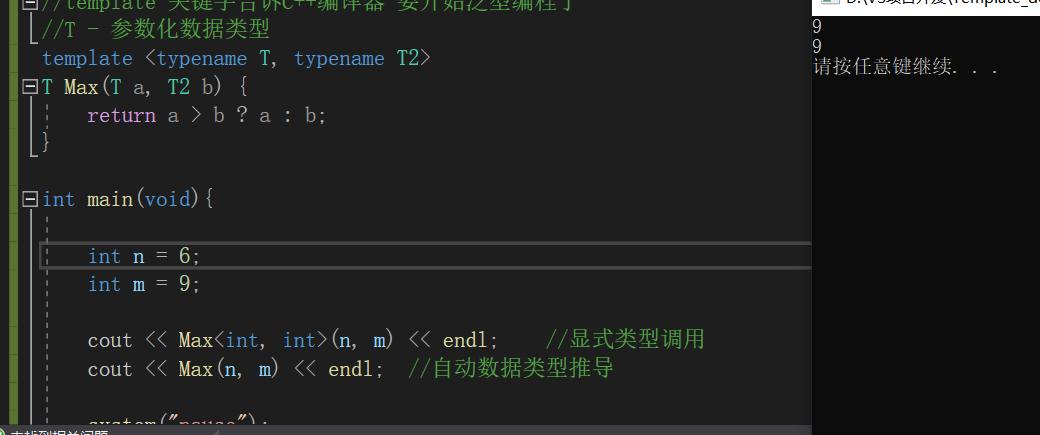我们再来看以下代码

#include <iostream>

using namespace std;

//template 关键字告诉C++编译器 要开始泛型编程了
//T - 参数化数据类型
template <typename T, typename T2>
T Max(T a, T2 b) {
return a > b ? a : b;
}

int main(void){

int n = 6;
int m = 9;
char a = 'c';	//'c' 对应的ascll码值是 99

cout << "Max<int, char>(m, a): " << Max<int, char>(m, a) << endl;	//显式类型调用
cout << "Max(m, a): " << Max(m, a) << endl;	//自动数据类型推导

cout << "Max(a, m): " << Max(a, m) << endl;	//自动数据类型推导

return 0;
}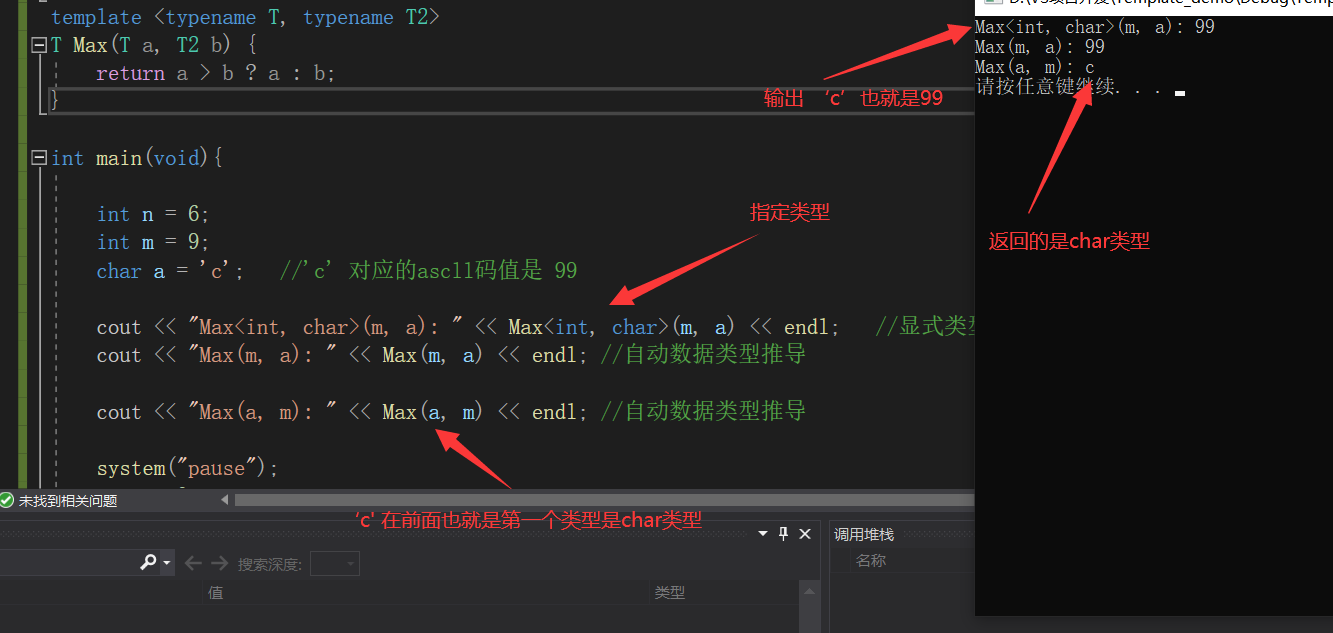为什么会这样呢？因为我们在定义函数模板的时候第一个类型作为了函数类型。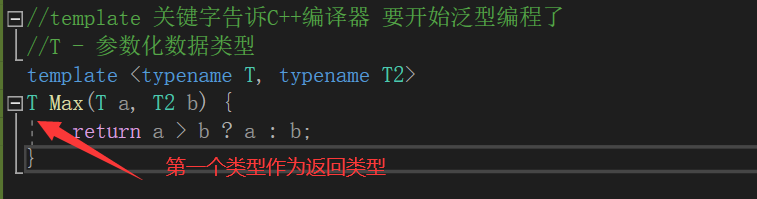# 模板函数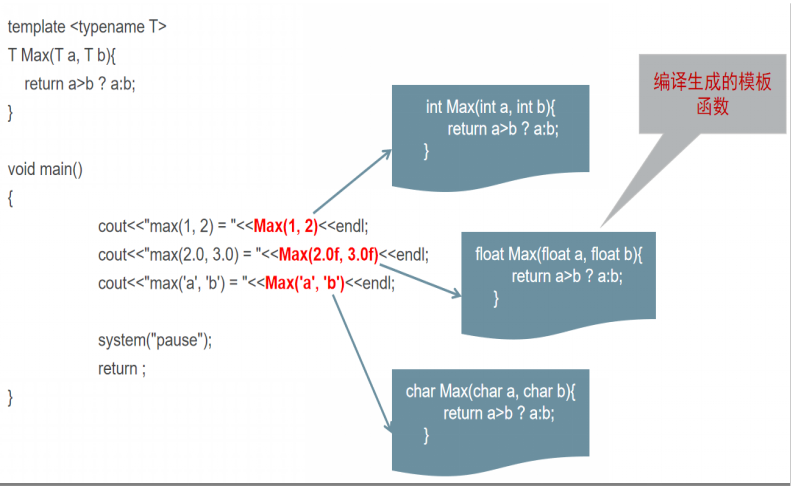也就是，在我们编译的时候，编译器内部会自动生成这些模板函数，然后再进行调用，当然，这些都是编译器内部生成的，我们可以不用去了解太深，我们知道这个概念就可以了。

如果我们来比较类的两个对象

#include <iostream>

using namespace std;

//定义一个类
class Son {
public:
Son(int age) { m_age = age; }
~Son(){}

int getAge() { return m_age; }

private:
int m_age;
};

//template 关键字告诉C++编译器 要开始泛型编程了
//T - 参数化数据类型
template <typename T, typename T2>
T Max(T a, T2 b) {
return a > b ? a : b;
}

int main(void){
Son s1(18);
Son s2(21);

cout << "Max(s1, s2): " << Max(s1, s2).getAge() << endl;
/*
* 模板函数 在类的对象中， 不认识 > 这个符号
Son Max(Son s1, Son s2){
return a > b ? a : b;
}
*/
return 0;
}


就会出现这样的错误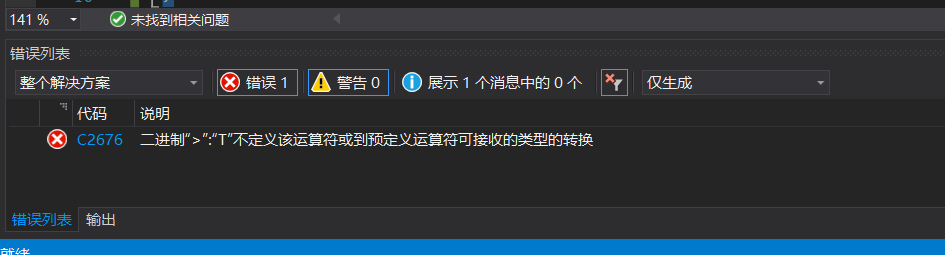因为在类对象的比较中，不认识这个 > 的符号，所以我们想要能成功执行，我们得在类中实现 > 的运算符重载

#include <iostream>

using namespace std;

//定义一个类
class Son {
public:
Son(int age) { m_age = age; }
~Son(){}

int getAge() { return m_age; }

//重载 > 运算符
bool operator >(Son& res) {
if (this->m_age > res.m_age) return true;

return false;
}

private:
int m_age;
};

//template 关键字告诉C++编译器 要开始泛型编程了
//T - 参数化数据类型
template <typename T, typename T2>
T Max(T a, T2 b) {
return a > b ? a : b;
}

int main(void){
Son s1(18);
Son s2(21);

cout << "Max(s1, s2): " << Max(s1, s2).getAge() << endl;
/*
* 模板函数 在类的对象中， 不认识 > 这个符号
Son Max(Son s1, Son s2){
return a > b ? a : b;
}
*/
return 0;
}


这样就可以执行得出结果了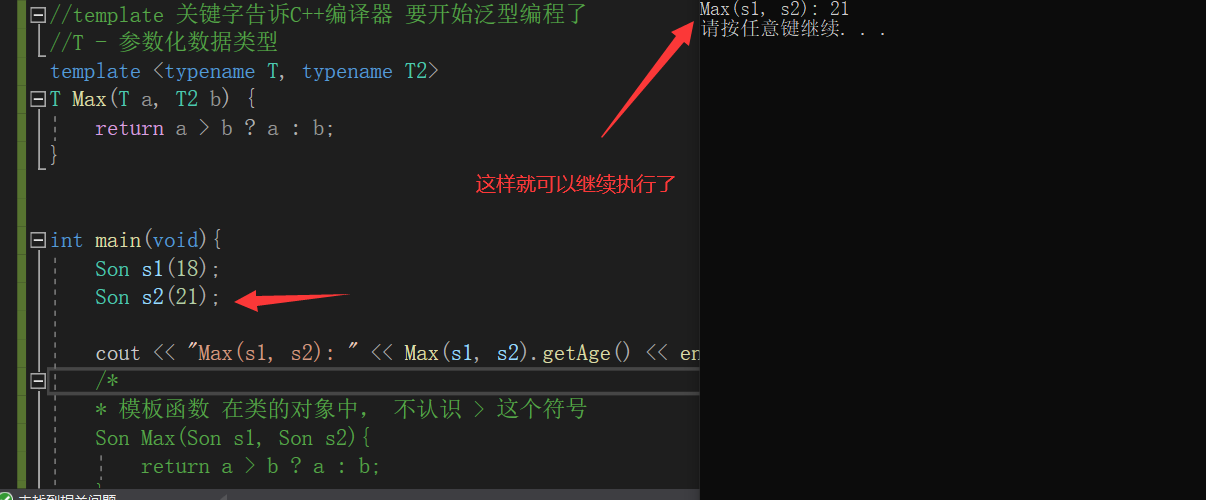# 函数模板和函数重载

我们先来看一下这个demo

#include <iostream>

using namespace std;

template <typename T>
void Test(T& a, T& b) {
T c;
c = a;
a = b;
b = c;

cout << "Test 函数模板被调用了.." << endl;
}

void Test(int& a, int& b) {
int c;
c = a;
a = b;
b = c;

cout << "Test 普通函数被调用.." << endl;
}

int main(void) {
int n = 99;
int m = 65;

Test(n, m);

return 0;
}


这是来观察，当存在函数模板和普通对应的函数的时候，会调用哪个函数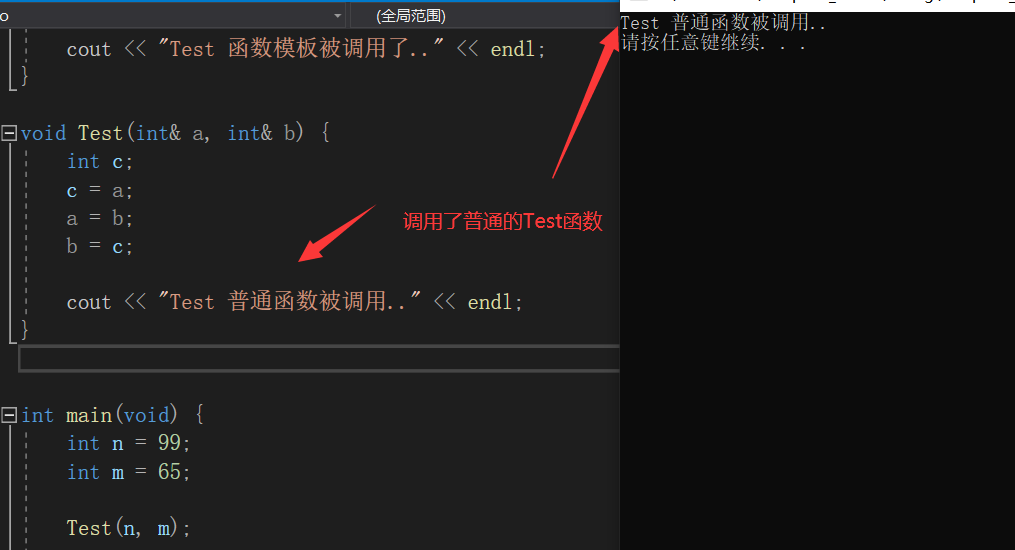可以看到 当函数模板和普通函数并存的时候，参数类型会和普通重载函数更加匹配

我们把普通函数注释掉，我们才能看到调用函数模板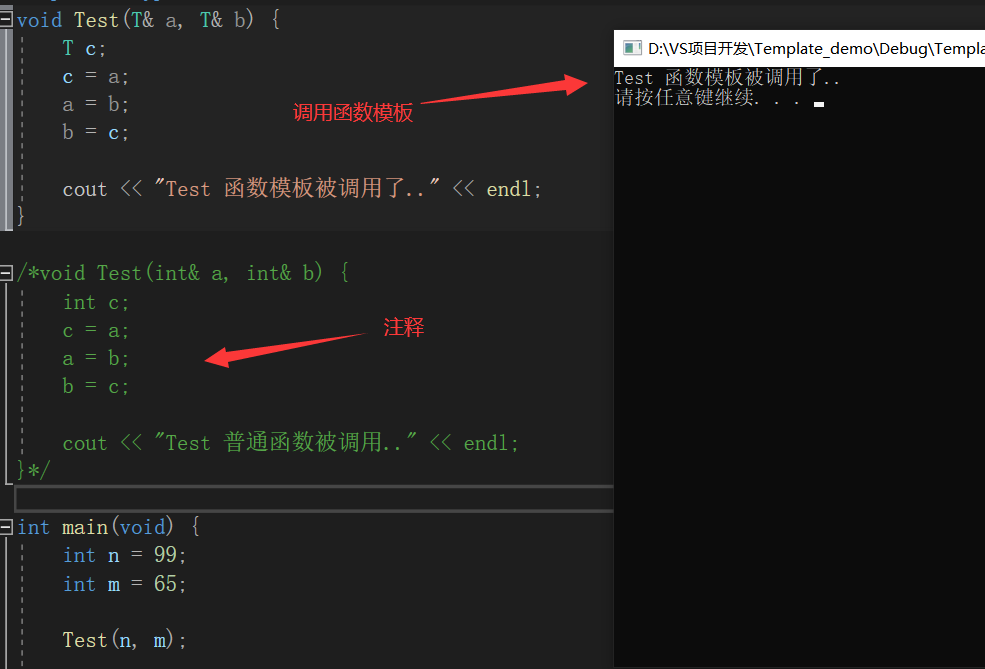如果普通函数和函数模板都在的情况下，我们非要使用函数模板，这个怎么实现呢，很简单，如果显式的使用函数模板,则使用<> 类型列表。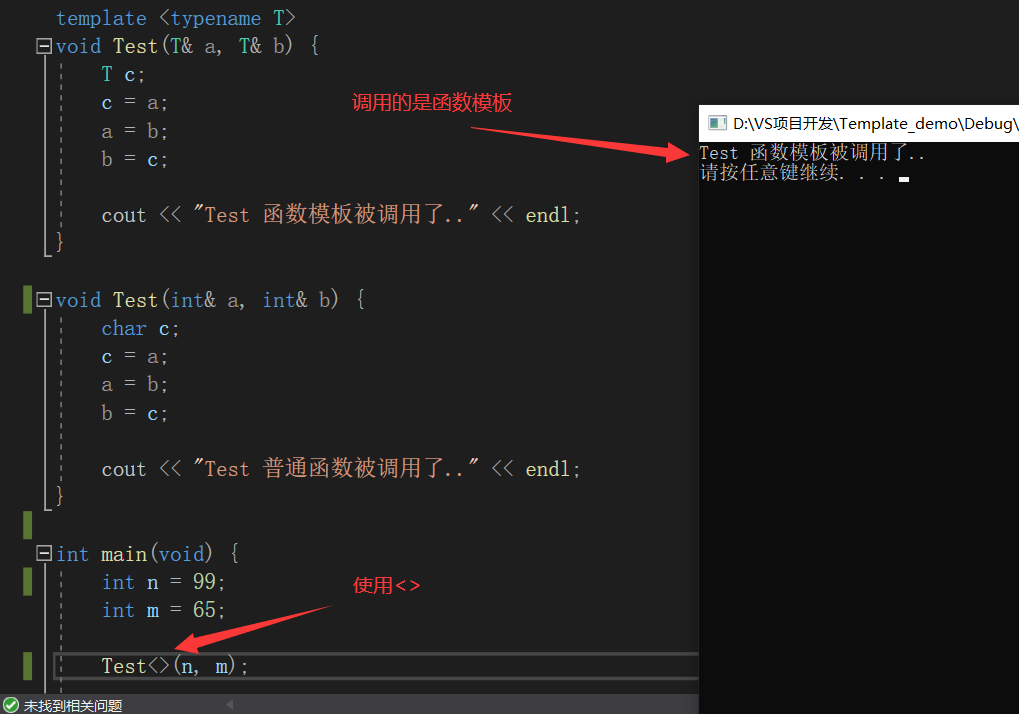如果我们将一个传递的参数改为别的类型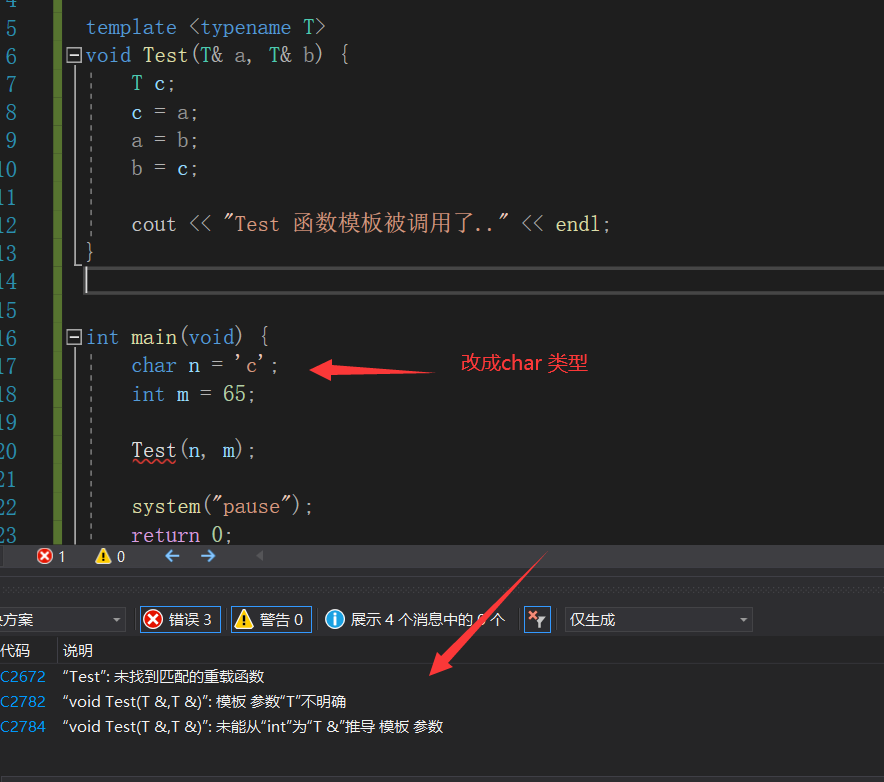不存在对应的普通函数，函数模板不会提供隐式的类型转换，必须是严格的类型匹配

函数模板和普通函数区别结论：

两者允许并存
函数模板不允许自动类型转化

如果有函数模板和普通函数的时候，普通函数的参数类型不不一致的时候，也会调用普通函数，因为会有隐式的转换，但是如果有函数模板的时候，函数模板会产生更好的匹配，使用函数模板。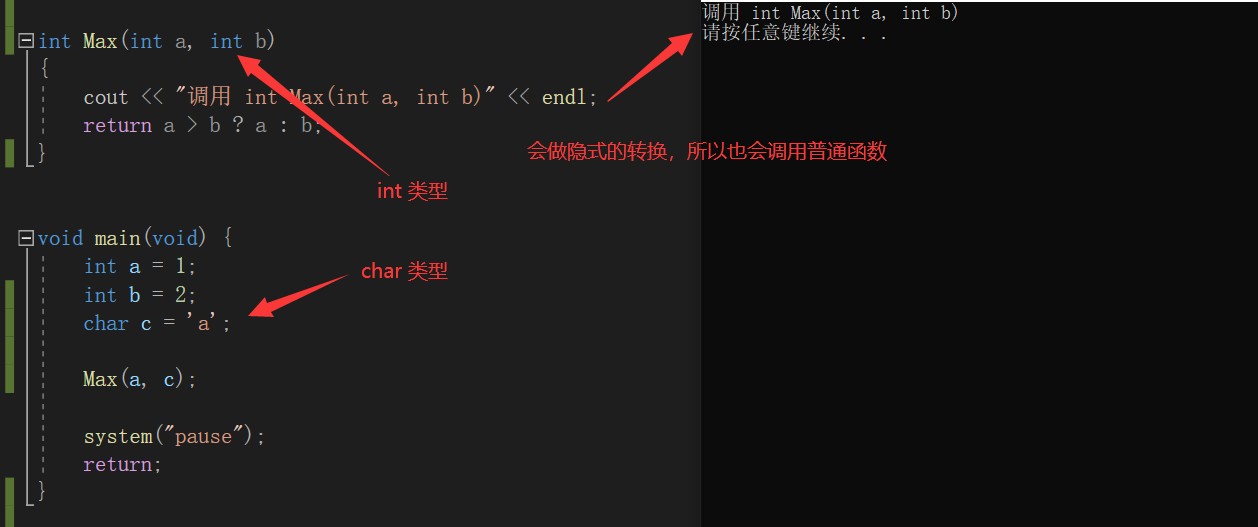有更好的函数模板的时候，会选择函数模板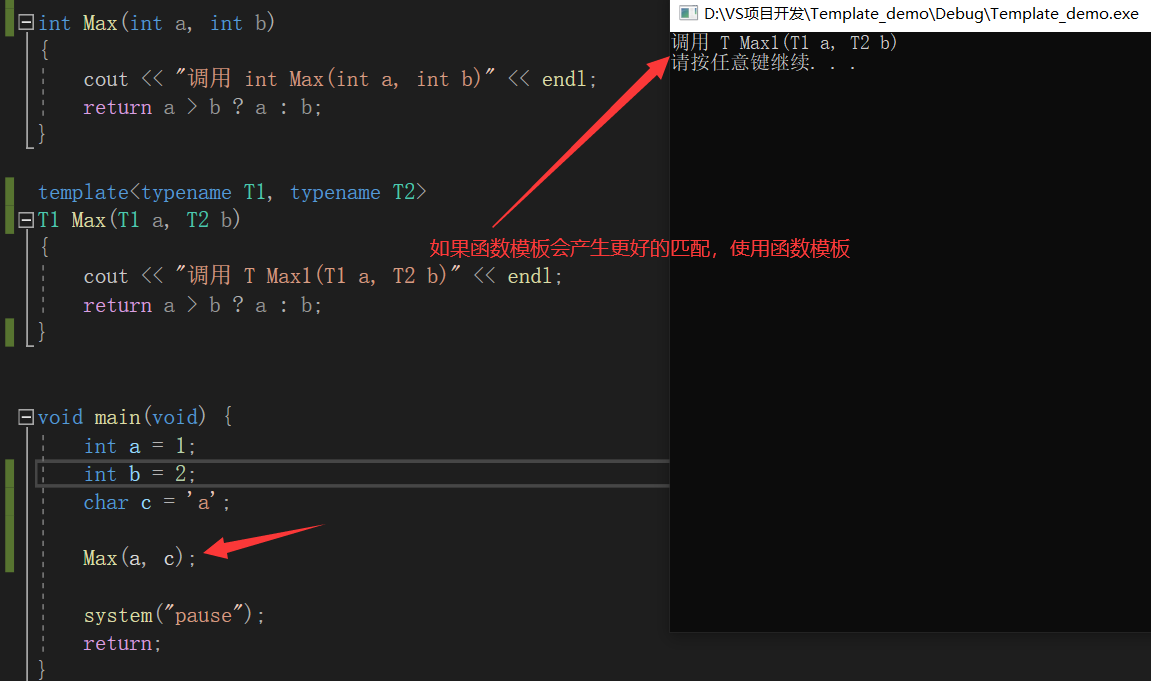## 嵌套使用函数模板

在函数模板中，我们也可以嵌套使用函数模板。

#include <iostream>

using namespace std;

template<typename T>
T Max(T a, T b)
{
cout << "调用 T Max(T a, T b)" << endl;
return a > b ? a : b;
}

template <typename T>
T Max(T a, T b, T c) {
cout << "调用 T Max(T a, T b, T c)" << endl;
return Max(Max(a, b), c);
}

int main(void) {
int a = 1;
int b = 2;
int c = 3;

Max(a, b, c);

system("pause");
return 0;
}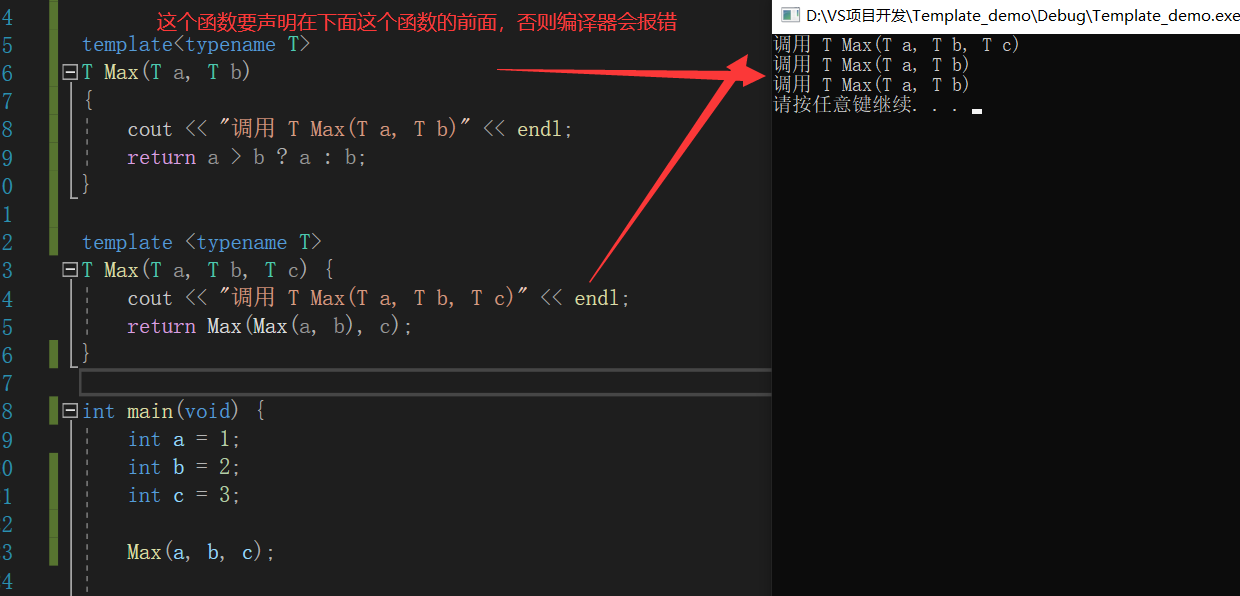注意：嵌套中的函数要声明在这个函数之前，否则编译器会找不到哪个函数，然后会报错

# 函数模板和普通函数在一起，调用规则

1 函数模板可以像普通函数一样被重载
2 C++编译器优先考虑普通函数
3 如果函数模板可以产生一个更好的匹配，那么选择模板
4 可以通过空模板实参列表的语法限定编译器只通过模板匹配

# 在Linux中反汇编查看函数模板被调用的机制

在Linux创建一个cpp文件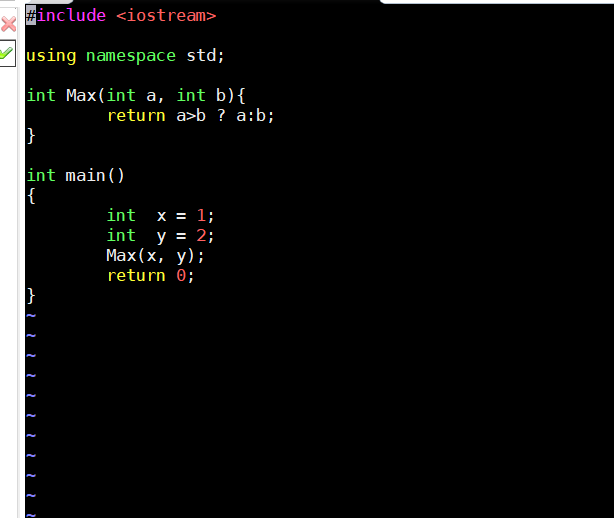保存退出之后编译生成指定的汇编的文件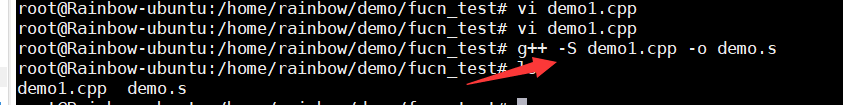然后查看这个汇编文件，找到我们熟悉的main函数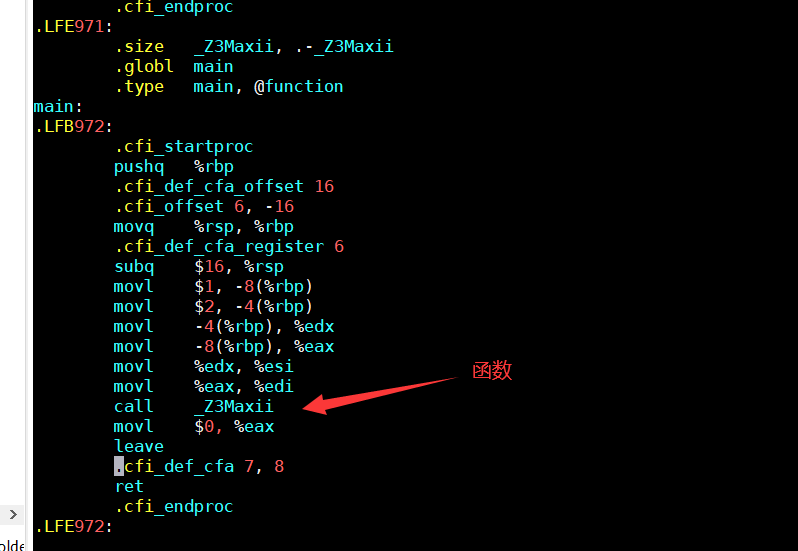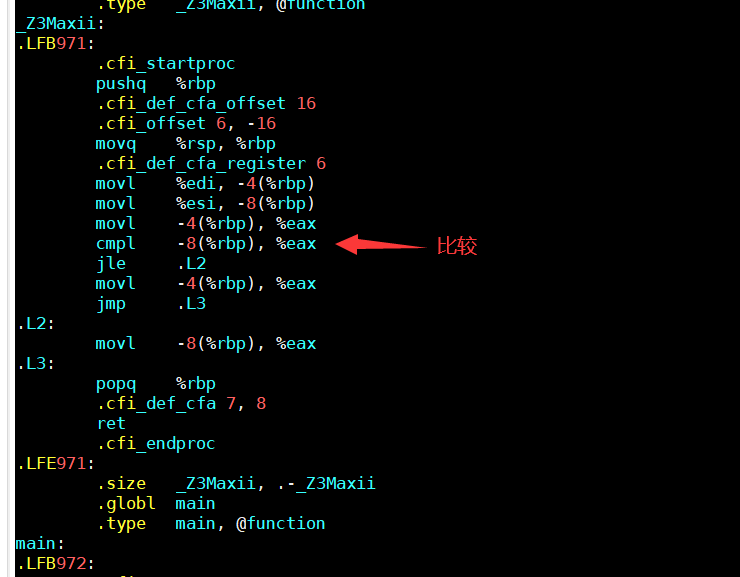再执行一个有函数模板的CPP文件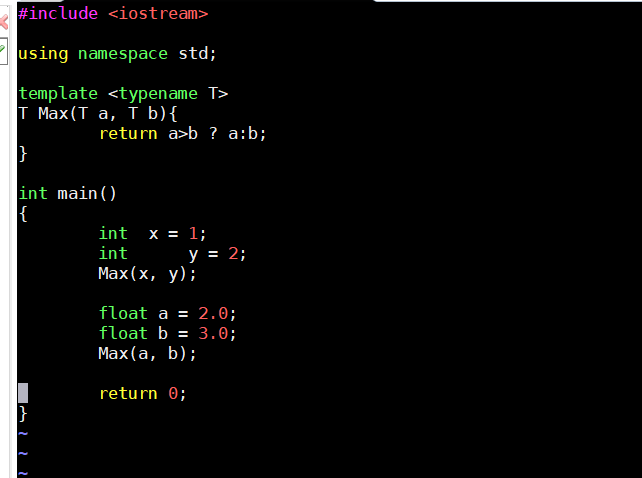在main函数中调用了函数模板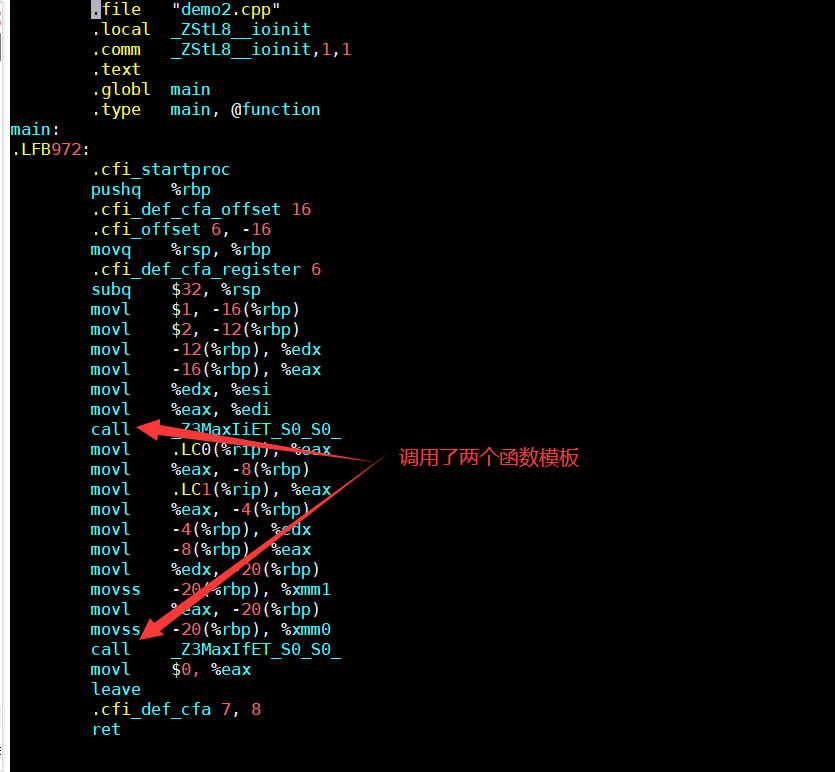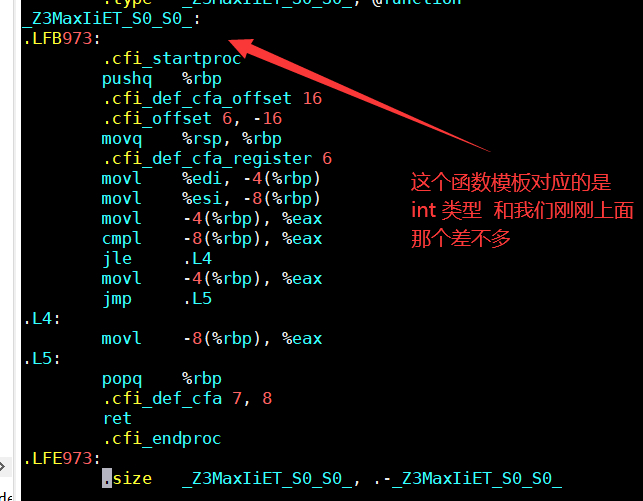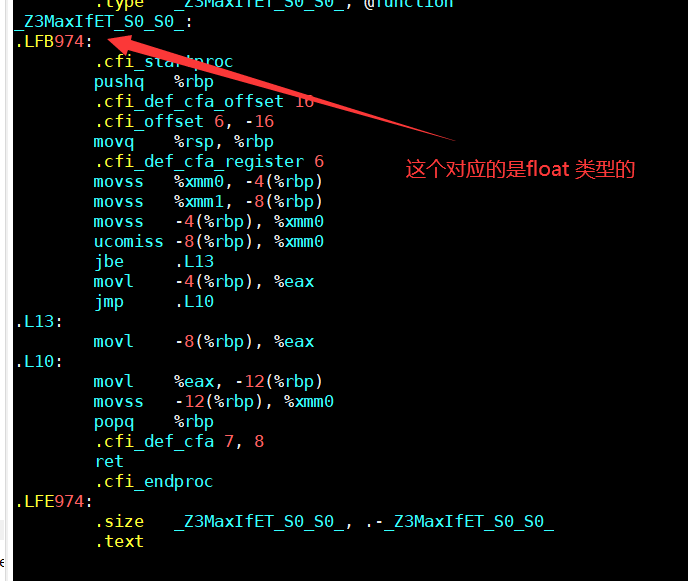从上面可以看出，我们只是定义了一个函数模板，而在调用的时候，内部会自动帮我们生成一个模板函数，然后再执行这个模板函数，得到我们想要的结果

结论

1. 编译器并不是把函数模板处理成能够处理任意类型的函数
2. 编译器从函数模板通过具体类型产生不同的函数
展开全文linux
• ## C++函数模板（模板函数）详解

万次阅读 多人点赞 2019-07-04 16:03:01
C++函数模板（模板函数）详解定义用法：函数模板的原理延申用法2.1为什么需要类模板2.2单个类模板语法2.3继承中的类模板语法案例1：案例2：2.4类模板的基础语法2.5类模板语法知识体系梳理1.所有的类模板函数写在类的...

# 定义

函数模板不是一个实在的函数，编译器不能为其生成可执行代码。定义函数模板后只是一个对函数功能框架的描述，当它具体执行时，将根据传递的实际参数决定其功能。（好吧，咱也听不懂，直接上用法吧?）

# 用法：

面向对象的继承和多态机制有效提高了程序的可重用性和可扩充性。在程序的可重用性方面，程序员还希望得到更多支持。举一个最简单的例子，为了交换两个整型变量的值，需要写下面的 Swap 函数：

void Swap(int & x, int & y)
{
int tmp = x;
x = y;
y = tmp;
}


为了交换两个 double 型变量的值，还需要编写下面的 Swap 函数：

void Swap (double & xr double & y)
{
double tmp = x;
x = y;
y = tmp;
}


如果还要交换两个 char 型变量的值，交换两个 CStudent 类对象的值……都需要再编写 Swap 函数。而这些 Swap 函数除了处理的数据类型不同外，形式上都是一样的。能否只写一遍 Swap 函数，就能用来交换各种类型的变量的值呢？继承和多态显然无法解决这个问题。因此，“模板”的概念就应运而生了。

众所周知，有了“模子”后，用“模子”来批量制造陶瓷、塑料、金属制品等就变得容易了。程序设计语言中的模板就是用来批量生成功能和形式都几乎相同的代码的。有了模板，编译器就能在需要的时候，根据模板自动生成程序的代码。从同一个模板自动生成的代码，形式几乎是一样的。

# 函数模板的原理

C++ 语言支持模板。有了模板，可以只写一个 Swap 模板，编译器会根据 Swap 模板自动生成多个 Sawp 函数，用以交换不同类型变量的值。

在 C++ 中，模板分为函数模板和类模板两种。

• 函数模板是用于生成函数；
• 类模板则是用于生成类的。

函数模板的写法如下：

template <class 类型参数1, class类型参数2, ...>
返回值类型  模板名(形参表)
{
函数体
}


其中的 class 关键字也可以用 typename 关键字替换，例如：

template <typename 类型参数1, typename 类型参数2, ...>


函数模板看上去就像一个函数。前面提到的 Swap 模板的写法如下：

template <class T>
void Swap(T & x, T & y)
{
T tmp = x;
x = y;
y = tmp;
}


T 是类型参数，代表类型。编译器由模板自动生成函数时，会用具体的类型名对模板中所有的类型参数进行替换，其他部分则原封不动地保留。同一个类型参数只能替换为同一种类型。编译器在编译到调用函数模板的语句时，会根据实参的类型判断该如何替换模板中的类型参数。

例如下面的程序：

#include <iostream>
using namespace std;
template<class T>
void Swap(T & x, T & y)
{
T tmp = x;
x = y;
y = tmp;
}
int main()
{
int n = 1, m = 2;
Swap(n, m);  //编译器自动生成 void Swap (int &, int &)函数
double f = 1.2, g = 2.3;
Swap(f, g);  //编译器自动生成 void Swap (double &, double &)函数
return 0;
}


编译器在编译到Swap(n, m);时找不到函数 Swap 的定义，但是发现实参 n、m 都是 int 类型的，用 int 类型替换 Swap 模板中的 T 能得到下面的函数：

void Swap (int & x, int & y)
{
int tmp = x;
x = y;
y = tmp;
}


该函数可以匹配Swap(n, m);这条语句。于是编译器就自动用 int 替换 Swap 模板中的 T，生成上面的 Swap 函数，将该 Swap 函数的源代码加入程序中一起编译，并且将Swap(n, m);编译成对自动生成的 Swap 函数的调用。

同理，编译器在编译到Swap(f, g);时会用 double 替换 Swap 模板中的 T，自动生成以下 Swap 函数：

void Swap(double & x, double & y)
{
double tmp = x;
x = y;
y = tmp;
}


然后再将Swap(f, g);编译成对该 Swap 函数的调用。

编译器由模板自动生成函数的过程叫模板的实例化。由模板实例化而得到的函数称为模板函数。在某些编译器中，模板只有在被实例化时，编译器才会检查其语法正确性。如果程序中写了一个模板却没有用到，那么编译器不会报告这个模板中的语法错误。

编译器对模板进行实例化时，并非只能通过模板调用语句的实参来实例化模板中的类型参数，模板调用语句可以明确指明要把类型参数实例化为哪种类型。可以用：

模板名<实际类型参数1, 实际类型参数2, ...>


的方式告诉编译器应该如何实例化模板函数。例如下面的程序：

#include <iostream>
using namespace std;
template <class T>
T Inc(int n)
{
return 1 + n;
}
int main()
{
cout << Inc<double>(4) / 2;
return 0;
}


Inc(4)指明了此处实例化的模板函数原型应为：

double Inc(double);


编译器不会因为实参 4 是 int 类型，就生成原型为 int Inc(int) 的函数。因此，上面程序输出的结果是 2.5 而非 2。

函数模板中可以有不止一个类型参数。例如，下面这个函数模板的写法是合法的：

template <class Tl, class T2>
T2 print(T1 argl, T2 arg2)
{
cout << arg1 << " " << arg2 << endl;
return arg2;
}


【实例】一个求数组中最大元素的函数模板
例题：设计一个分数类 CFraction，再设计一个名为 MaxElement 的函数模板，能够求数组中最大的元素，并用该模板求一个 CFmction 数组中的最大元素。

示例程序如下：

#include <iostream>
using namespace std;
template <class T>
T MaxElement(T a[], int size) //size是数组元素个数
{
T tmpMax = a;
for (int i = 1; i < size; ++i)
if (tmpMax < a[i])
tmpMax = a[i];
return tmpMax;
}
class CFraction //分数类
{
int numerator;   //分子
int denominator; //分母
public:
CFraction(int n, int d) :numerator(n), denominator(d) { };
bool operator <(const CFraction & f) const
{//为避免除法产生的浮点误差，用乘法判断两个分数的大小关系
if (denominator * f.denominator > 0)
return numerator * f.denominator < denominator * f.numerator;
else
return numerator * f.denominator > denominator * f.numerator;
}
bool operator == (const CFraction & f) const
{//为避免除法产生的浮点误差，用乘法判断两个分数是否相等
return numerator * f.denominator == denominator * f.numerator;
}
friend ostream & operator <<(ostream & o, const CFraction & f);
};
ostream & operator <<(ostream & o, const CFraction & f)
{//重载 << 使得分数对象可以通过cout输出
o << f.numerator << "/" << f.denominator; //输出"分子/分母" 形式
return o;
}
int main()
{
int a = { 1,5,2,3,4 };
CFraction f = { CFraction(8,6),CFraction(-8,4),
CFraction(3,2), CFraction(5,6) };
cout << MaxElement(a, 5) << endl;
cout << MaxElement(f, 4) << endl;
return 0;
}


编译到第 41 行时，根据实参 a 的类型，编译器通过 MaxElement 模板自动生成了一个 MaxElement 函数，原型为：

int MaxElement(int a[], int size);


编译到第 42 行时，根据 f 的类型，编译器又生成一个 MaxElement 函数，原型为：

CFraction MaxElement(CFraction a[], int size);


在该函数中，用到了<比较两个 CFraction 对象的大小。如果没有对<进行适当的重载，编译时就会出错。

从 MaxElement 模板的写法可以看出，在函数模板中，类型参数不但可以用来定义参数的类型，还能用于定义局部变量和函数模板的返回值。

# 延申用法

## 2.1为什么需要类模板

• 类模板与函数模板的定义和使用类似，我们已经进行了介绍。 有时，有两个或多个类，其功能是相同的，仅仅是数据类型不同，如下面语句声明了一个类：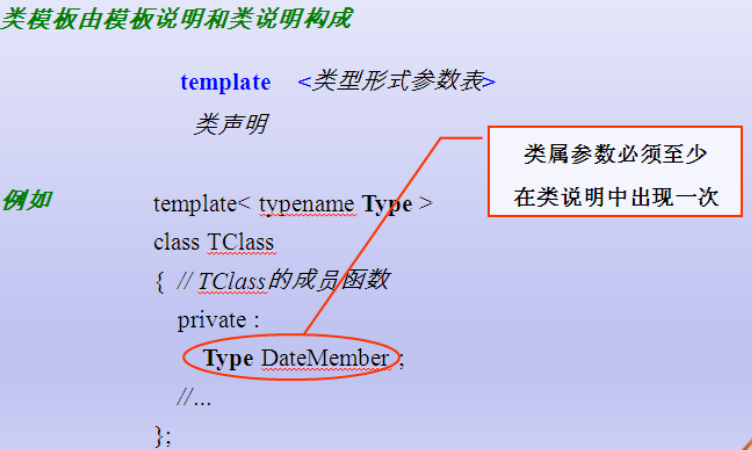• 类模板用于实现类所需数据的类型参数化

• 类模板在表示如数组、表、图等数据结构显得特别重要，

• 这些数据结构的表示和算法不受所包含的元素类型的影响

## 2.2单个类模板语法

 1 //类的类型参数化 抽象的类
2 //单个类模板
3 template<typename T>
4 class A
5 {
6 public:
7     A(T t)
8     {
9         this->t = t;
10     }
11
12     T &getT()
13     {
14         return t;
15     }
16 protected:
17 public:
18     T t;
19 };
20 void main()
21 {
22    //模板了中如果使用了构造函数,则遵守以前的类的构造函数的调用规则
23     A<int>  a(100);
24     a.getT();
25     printAA(a);
26     return ;
27 }


## 2.3继承中的类模板语法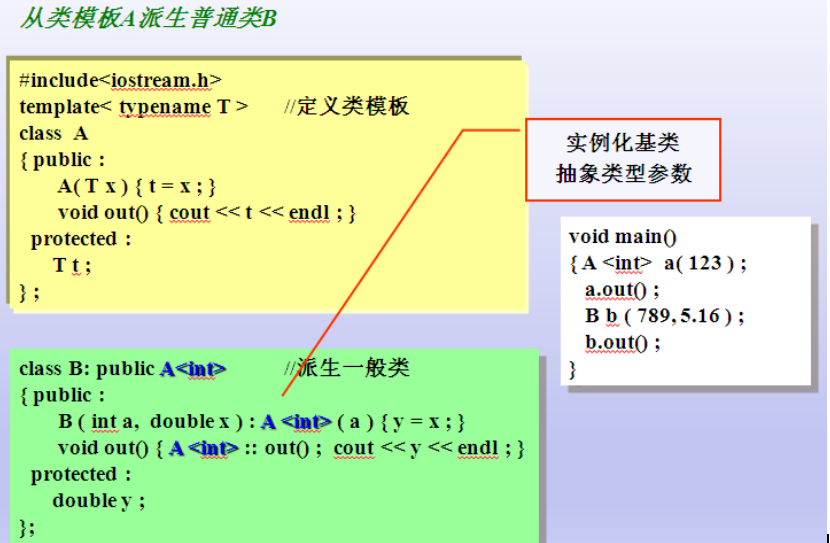### 案例1：

 1 //结论: 子类从模板类继承的时候,需要让编译器知道 父类的数据类型具体是什么(数据类型的本质:固定大小内存块的别名)A<int>
2 //
3 class B : public A<int>
4 {
5 public:
6     B(int i) : A<int>(i)
7     {
8
9     }
10     void printB()
11     {
12         cout<<"A:"<<t<<endl;
13     }
14 protected:
15 private:
16 };
17
18 //模板与上继承
19 //怎么样从基类继承
20 //若基类只有一个带参数的构造函数,子类是如何启动父类的构造函数
21 void pintBB(B &b)
22 {
23     b.printB();
24 }
25 void printAA(A<int> &a)  //类模板做函数参数
26 {
27      //
28     a.getT();
29 }
30
31 void main()
32 {
33     A<int>  a(100); //模板了中如果使用了构造函数,则遵守以前的类的构造函数的调用规则
34     a.getT();
35     printAA(a);
36
37     B b(10);
38     b.printB();
39
40
41     cout<<"hello..."<<endl;
42     system("pause");
43     return ;
44 }


### 案例2：

 1 #include<iostream>
2 using namespace std;
3 //A编程模板类--类型参数化
4 /*
5 类模板的定义 类模板的使用 类模板做函数参数
6 */
7 template <typename T>
8 class A
9 {
10 public:
11     A(T a = 0)
12     {
13         this->a = a;
14     }
15 public:
16     void printA()
17     {
18         cout << "a:" << a << endl;
19     }
20 protected:
21     T a;
22 private:
23
24 };
25 //从模板类派生时，需要具体化模板类，C++编译器需要知道父类的数据类型是什么样子的
26 //要知道父类所占的内存多少
27 class B :public A<int>
28 {
29 public:
30     B(int a =10, int b =20):A<int>(a)
31     {
32         this->b = b;
33     }
34     void printB()
35     {
36         cout << "a:" << a << "b:" << b << endl;
37     }
38 protected:
39 private:
40     int b;
41
42 };
43 //从模板类派生模板类
44 template <typename T>
45 class C :public A<T>
46 {
47
48 public:
49     C(T c,T a) : A<T>(a)
50     {
51         this->c = c;
52     }
53     void printC()
54     {
55         cout << "c:" << c << endl;
56     }
57 protected:
58     T c;
59 private:
60
61 };
62
63 void main()
64 {
65     //B b1(1, 2);
66     //b1.printB();
67     C<int> c1(1,2);
68     c1.printC();
69 }


## 2.4类模板的基础语法

 1 #include<iostream>
2 using namespace std;
3 //A编程模板类--类型参数化
4 /*
5     类模板的定义 类模板的使用 类模板做函数参数
6 */
7 template <typename T>
8 class A
9 {
10 public:
11     A(T a = 0)
12     {
13         this->a = a;
14     }
15 public:
16     void printA()
17     {
18         cout << "a:" << a << endl;
19     }
20 protected:
21 private:
22     T a;
23 };
24 //参数 C++编译器具体的类
25 void UseA(A<int> &a)
26 {
27     a.printA();
28 }
29 void main()
30 {
31     //模板类本身就是抽象的，具体的类，具体的变量
32     A<int> a1(11),a2(22),a3(33);//模板类是抽象的， 需要类型具体化
33     //a1.printA();
34
35     UseA(a1);
36     UseA(a2);
37     UseA(a3);
38 }


## 2.5类模板语法知识体系梳理

### 1.所有的类模板函数写在类的内部

代码：

#### 复数类：

 1 #include<iostream>
2 using namespace std;
3 template <typename T>
4 class Complex
5 {
6 public:
7     friend Complex MySub(Complex &c1, Complex &c2)
8     {
9         Complex tmp(c1.a-c2.a, c1.b-c2.b);
10         return tmp;
11     }
12
13     friend ostream & operator<< (ostream &out, Complex &c3)
14     {
15         out << c3.a << "+" << c3.b <<"i"<< endl;
16         return out;
17     }
18     Complex(T a, T b)
19     {
20         this->a = a;
21         this->b = b;
22     }
23     Complex operator+(Complex &c2)
24     {
25         Complex tmp(a + c2.a, b + c2.b);
26         return tmp;
27     }
28     void printCom()
29     {
30         cout << "a:" << a << " b:" << b << endl;
31     }
32 protected:
33 private:
34     T a;
35     T b;
36 };
37
38 /*
39     重载运算符的正规写法：
40     重载左移<<  右移>> 只能用友元函数，其他的运算符重载都要用成员函数，不要滥用友元函数
41 */
42 //ostream & operator<< (ostream &out, Complex &c3)
43 //{
44 //    out<< "a:" << c3.a << " b:" << c3.b << endl;
45 //    return out;
46 //}
47 void main()
48 {
49     Complex<int>     c1(1,2);
50     Complex<int>     c2(3, 4);
51
52     Complex<int> c3 = c1 + c2;//重载加号运算符
53
54     c3.printCom();
55
56     //重载左移运算符
57     cout << c3 << endl;
58
59     {
60         Complex<int> c4 = MySub(c1 , c2);
61
62         cout << c4 << endl;
63     }
64     system("pause");
65 }


### 2.所有的类模板函数写在类的外部，在一个cpp中

注意：

复制代码
//构造函数 没有问题

• 普通函数 没有问题

• 友元函数：用友元函数重载 << >>

friend ostream& operator<< (ostream &out, Complex &c3) ;

• 友元函数：友元函数不是实现函数重载（非 << >>）

1 需要在类前增加 类的前置声明 函数的前置声明

template<typename T>

class Complex;

template<typename T>

Complex<T> mySub(Complex<T> &c1, Complex<T> &c2);


2 类的内部声明 必须写成:

friend Complex<T> mySub <T> (Complex<T> &c1, Complex<T> &c2);


3 友元函数实现 必须写成：

template<typename T>

Complex<T> mySub(Complex<T> &c1, Complex<T> &c2)

{

Complex<T> tmp(c1.a - c2.a, c1.b-c2.b);

return tmp;

}


4 友元函数调用 必须写成

Complex<int> c4 = mySub<int>(c1, c2)；

cout<<c4;


结论：友元函数只用来进行 左移 友移操作符重载。

复数类：

代码：

 1 #include<iostream>
2 using namespace std;
3
4 template<typename T>
5 class Complex;
6 template<typename T>
7 Complex<T> mySub(Complex<T> &c1, Complex<T> &c2);
8
9 template <typename T>
10 class Complex
11 {
12 public:
13     friend Complex<T> mySub <T>(Complex<T> &c1, Complex<T> &c2);
14
15     friend ostream & operator<< <T>(ostream &out, Complex &c3);
16     Complex(T a, T b);
17     void printCom();
18     Complex operator+(Complex &c2);
19     Complex operator-(Complex &c2);
20
21 protected:
22 private:
23     T a;
24     T b;
25 };
26
27 //构造函数的实现，写在了外部
28 template <typename T>
29 Complex<T>::Complex(T a, T b)
30 {
31     this->a = a;
32     this->b = b;
33 }
34
35 template <typename T>
36 void Complex<T>::printCom()
37 {
38     cout << "a:" << a << " b:" << b << endl;
39 }
40 //成员函数实现加号运算符重载
41 template <typename T>
42 Complex<T> Complex<T>::operator+(Complex<T> &c2)
43 {
44     Complex tmp(a + c2.a, b + c2.b);
45     return tmp;
46 }
47 template <typename T>
48 Complex<T> Complex<T>::operator-(Complex<T> &c2)
49 {
50     Complex(a-c2.a,a-c2.b);
51     return tmp;
52 }
53 //友元函数实现<<左移运算符重载
54
55 /*
56 严重性    代码    说明    项目    文件    行    禁止显示状态
57 错误    C2768    “operator <<”: 非法使用显式模板参数    泛型编程课堂操练
58
59 错误的本质：两次编译的函数头，第一次编译的函数头，和第二次编译的函数有不一样
60 */
61 template <typename T>
62 ostream & operator<< (ostream &out, Complex<T> &c3)//不加T
63 {
64     out << c3.a << "+" << c3.b << "i" << endl;
65     return out;
66 }
67
68
69 //
70 template <typename T>
71 Complex<T> mySub(Complex<T> &c1, Complex<T> &c2)
72 {
73     Complex<T> tmp(c1.a - c2.a, c1.b - c2.b);
74     return tmp;
75 }
76
77 void main()
78 {
79     Complex<int>     c1(1, 2);
80     Complex<int>     c2(3, 4);
81
82     Complex<int> c3 = c1 + c2;//重载加号运算符
83
84     c3.printCom();
85
86     //重载左移运算符
87     cout << c3 << endl;
88
89     {
90         Complex<int> c4 = mySub<int>(c1, c2);
91
92         cout << c4 << endl;
93     }
94     system("pause");
95 }


所有的类模板函数写在类的外部，在不同的.h和.cpp中
也就是类模板函数说明和类模板实现分开

//类模板函数

构造函数

普通成员函数

友元函数

用友元函数重载<<>>;

用友元函数重载非<< >>

demo_complex.cpp

 1 #include"demo_09complex.h"
2 #include<iostream>
3 using namespace std;
4
5 template <typename T>
6 Complex<T>::Complex(T a, T b)
7 {
8     this->a = a;
9     this->b = b;
10 }
11
12 template <typename T>
13 void Complex<T>::printCom()
14 {
15     cout << "a:" << a << " b:" << b << endl;
16 }
17 //成员函数实现加号运算符重载
18 template <typename T>
19 Complex<T> Complex<T>::operator+(Complex<T> &c2)
20 {
21     Complex tmp(a + c2.a, b + c2.b);
22     return tmp;
23 }
24 //template <typename T>
25 //Complex<T> Complex<T>::operator-(Complex<T> &c2)
26 //{
27 //    Complex(a - c2.a, a - c2.b);
28 //    return tmp;
29 //}
30 template <typename T>
31 ostream & operator<< (ostream &out, Complex<T> &c3)//不加T
32 {
33     out << c3.a << "+" << c3.b << "i" << endl;
34     return out;
35 }
36
37
38 //
39 //template <typename T>
40 //Complex<T> mySub(Complex<T> &c1, Complex<T> &c2)
41 //{
42 //    Complex<T> tmp(c1.a - c2.a, c1.b - c2.b);
43 //    return tmp;
44 //}


demo_09complex.h

 1 #pragma once
2 #include<iostream>
3 using namespace std;
4 template <typename T>
5 class Complex
6 {
7 public:
8     //friend Complex<T> mySub <T>(Complex<T> &c1, Complex<T> &c2);
9
10     friend ostream & operator<< <T>(ostream &out, Complex &c3);
11     Complex(T a, T b);
12     void printCom();
13     Complex operator+(Complex &c2);
14     //Complex operator-(Complex &c2);
15
16 protected:
17 private:
18     T a;
19     T b;
20 };


demo_09complex_text.cpp

 1 #include"demo_09complex.h"
2 #include"demo_09complex.cpp"
3
4 #include<iostream>
5 using namespace std;
6
7 void main()
8 {
9     Complex<int>     c1(1, 2);
10     Complex<int>     c2(3, 4);
11
12     Complex<int> c3 = c1 + c2;//重载加号运算符
13
14     c3.printCom();
15
16     //重载左移运算符
17     cout << c3 << endl;
18
19     /*{
20         Complex<int> c4 = mySub<int>(c1, c2);
21
22         cout << c4 << endl;
23     }*/
24     system("pause");
25 }


## 2.5总结

归纳以上的介绍，可以这样声明和使用类模板：

1. 先写出一个实际的类。由于其语义明确，含义清楚，一般不会出错。
2. 将此类中准备改变的类型名(如int要改变为float或char)改用一个自己指定的虚拟类型名(如上例中的numtype)。
3. 在类声明前面加入一行，格式为：

  template <class 虚拟类型参数>

如：

template <class numtype> //注意本行末尾无分号

class Compare

{…}; //类体

1. 用类模板定义对象时用以下形式：

 类模板名<实际类型名> 对象名;

类模板名<实际类型名> 对象名(实参表列);

如：

Compare<int> cmp;

Compare<int> cmp(3,7);

1. 如果在类模板外定义成员函数，应写成类模板形式：

 template <class 虚拟类型参数>

函数类型 类模板名<虚拟类型参数>::成员函数名(函数形参表列) {…}


### 关于类模板的几点说明：

1. 类模板的类型参数可以有一个或多个，每个类型前面都必须加class，如：

template <class T1,class T2>

class someclass

{…};


在定义对象时分别代入实际的类型名，如：

someclass<int,double> obj;

2. 和使用类一样，使用类模板时要注意其作用域，只能在其有效作用域内用它定义对象。

3. 模板可以有层次，一个类模板可以作为基类，派生出派生模板类。

## 2.6类模板中的static关键字

从类模板实例化的每个模板类有自己的类模板数据成员，该模板类的所有对象共享一个static数据成员和非模板类的static数据成员一样，模板类的static数据成员也应该在文件范围定义和初始化
每个模板类有自己的类模板的static数据成员副本

 1 #include<iostream>
2 using namespace std;
3 template <typename T>
4 class AA
5 {
6 public:
7     static T m_a;
8 protected:
9 private:
10 };
11 template <typename T>
12 T AA<T>::m_a =0;
13 void main()
14 {
15     AA<int> a1, a2, a3;
16     a1.m_a = 10;
17     a2.m_a++;
18     a3.m_a++;
19     cout << AA<int>::m_a << endl;
20
21     AA<char> b1, b2, b3;
22     b1.m_a = 'a';
23     b2.m_a++;
24     b3.m_a++;
25     cout << AA<char>::m_a << endl;
26
27     //m_a是每个类型的类，去使用，手工写两个类 int  char
28     system("pause");
29 }


### 案例2：以下来自：C++类模板遇上static关键字

 1 #include <iostream>
2 using namespace std;
3
4 template<typename T>
5 class Obj{
6 public:
7     static T m_t;
8 };
9
10 template<typename T>
11 T Obj<T>::m_t = 0;
12
13 int main04(){
14     Obj<int> i1,i2,i3;
15     i1.m_t = 10;
16     i2.m_t++;
17     i3.m_t++;
18     cout << Obj<int>::m_t<<endl;
19
20     Obj<float> f1,f2,f3;
21     f1.m_t = 10;
22     f2.m_t++;
23     f3.m_t++;
24     cout << Obj<float>::m_t<<endl;
25
26     Obj<char> c1,c2,c3;
27     c1.m_t = 'a';
28     c2.m_t++;
29     c3.m_t++;
30     cout << Obj<char>::m_t<<endl;
31 }


当类模板中出现static修饰的静态类成员的时候，我们只要按照正常理解就可以了。static的作用是将类的成员修饰成静态的，所谓的静态类成员就是指类的成员为类级别的，不需要实例化对象就可以使用，而且类的所有对象都共享同一个静态类成员，因为类静态成员是属于类而不是对象。那么，类模板的实现机制是通过二次编译原理实现的。c++编译器并不是在第一个编译类模板的时候就把所有可能出现的类型都分别编译出对应的类（太多组合了），而是在第一个编译的时候编译一部分，遇到泛型不会替换成具体的类型（这个时候编译器还不知道具体的类型），而是在第二次编译的时候再将泛型替换成具体的类型（这个时候编译器知道了具体的类型了）。由于类模板的二次编译原理再加上static关键字修饰的成员，当它们在一起的时候实际上一个类模板会被编译成多个具体类型的类，所以，不同类型的类模板对应的static成员也是不同的（不同的类），但相同类型的类模板的static成员是共享的（同一个类）。

## 2.7类模板在项目开发中的应用

小结

• 模板是C++类型参数化的多态工具。C++提供函数模板和类模板。

• 模板定义以模板说明开始。类属参数必须在模板定义中至少出现一次。

• 同一个类属参数可以用于多个模板。

• 类属参数可用于函数的参数类型、返回类型和声明函数中的变量。

• 模板由编译器根据实际数据类型实例化，生成可执行代码。实例化的函数。

• 模板称为模板函数；实例化的类模板称为模板类。

• 函数模板可以用多种方式重载。

• 类模板可以在类层次中使用 。

## 训练题

### 1） 请设计一个数组模板类（ MyVector ），完成对int、char、Teacher类型元素的管理。

设计:

• 类模板 构造函数 拷贝构造函数 << [] 重载=操作符

• a2=a1

• 实现

### 2） 请仔细思考：

a) 如果数组模板类中的元素是Teacher元素时，需要Teacher类做什么工作

b) 如果数组模板类中的元素是Teacher元素时，Teacher类含有指针属性哪？

 1 class Teacher
2 {
3     friend ostream & operator<<(ostream &out, const Teacher &obj);
4 public:
5     Teacher(char *name, int age)
6     {
7         this->age = age;
8         strcpy(this->name, name);
9     }
10
11     Teacher()
12     {
13         this->age = 0;
14         strcpy(this->name, "");
15     }
16
17 private:
18     int age;
19     char name;
20 };
21
22
23 class Teacher
24 {
25     friend ostream & operator<<(ostream &out, const Teacher &obj);
26 public:
27     Teacher(char *name, int age)
28     {
29         this->age = age;
30         strcpy(this->name, name);
31     }
32
33     Teacher()
34     {
35         this->age = 0;
36         strcpy(this->name, "");
37     }
38
39 private:
40     int age;
41     char *pname;
42 };


结论1： 如果把Teacher放入到MyVector数组中，并且Teacher类的属性含有指针，就是出现深拷贝和浅拷贝的问题。

结论2：需要Teacher封装的函数有：

1. 重写拷贝构造函数

2. 重载等号操作符

3. 重载左移操作符。

理论提高：

所有容器提供的都是值（value）语意，而非引用（reference）语意。容器执行插入元素的操作时，内部实施拷贝动作。所以STL容器内存储的元素必须能够被拷贝（必须提供拷贝构造函数）。

### 3） 请从数组模板中进行派生

 1 //演示从模板类 派生 一般类
2 #include "MyVector.cpp"
3
4 class MyArray01 : public MyVector<double>
5 {
6 public:
7     MyArray01(int len) : MyVector<double>(len)
8     {
9         ;
10     }
11 protected:
12 private:
13 };
14
15
16 //演示从模板类 派生 模板类 //BoundArray
17 template <typename T>
18 class MyArray02 : public MyVector<T>
19 {
20 public:
21     MyArray02(int len) : MyVector<double>(len)
22     {
23         ;
24     }
25 protected:
26 private:
27 };
28 测试案例：
29
30 //演示 从模板类 继承 模板类
31 void main()
32 {
33     MyArray02<double> dArray2(10);
34     dArray2 = 3.15;
35
36 }
37
38
39 //演示 从模板类 继承 一般类
40 void main11()
41 {
42     MyArray01 d_array(10);
43
44     for (int i=0; i<d_array.getLen(); i++)
45     {
46         d_array[i] = 3.15;
47     }
48
49     for (int i=0; i<d_array.getLen(); i++)
50     {
51         cout << d_array[i] << " ";
52     }
53
54     cout<<"hello..."<<endl;
55     system("pause");
56     return ;
57 }


## 作业：

封装你自己的数组类；设计被存储的元素为类对象；

思考：类对象的类，应该实现的功能。

1. 优化Teacher类, 属性变成 char *panme, 构造函数里面 分配内存

2. 优化Teacher类,析构函数 释放panme指向的内存空间

3. 优化Teacher类,避免浅拷贝 重载= 重写拷贝构造函数

4. 优化Teacher类,在Teacher增加 <<

5. 在模板数组类中,存int char Teacher Teacher*(指针类型)

### zuoye.h

 1 #pragma once
2
3 template <typename T>
4 class MyVector
5 {
6
7     //friend ostream & operator<< <T>(ostream &out, const MyVector &obj);
8 public:
9     MyVector(int size = 0);//构造函数
10     MyVector(const MyVector &obj);//copy构造函数
11     ~MyVector();
12 public:
13     T& operator [](int index);
14     MyVector &operator=(const MyVector &obj);
15
16
17     int getLen()
18     {
19         return m_len;
20     }
21 protected:
22 private:
23     T *m_space;
24     int m_len;
25
26 };


### zuoye_test12.cpp

  1 #include"zuoye.h"
2 #include"zuoye12.cpp"
3 #include<iostream>
4 using namespace std;
5 class Teacher
6 {
7 public:
8     Teacher()
9     {
10         age = 33;
11         m_p = new char;
12         strcpy(m_p, " ");
13     }
14
15     Teacher(char *name, int age)
16     {
17         this->age = age;
18         m_p = new char[strlen(name)+1];
19         strcpy(this->m_p, name);
20
21     }
22     Teacher(const Teacher &obj)
23     {
24         m_p = new char[strlen(obj.m_p) + 1];
25         strcpy(this->m_p, obj.m_p);
26         age = obj.age;
27     }
28     ~Teacher()
29     {
30         if (m_p!=NULL)
31         {
32             delete[] m_p;
33             m_p = NULL;
34         }
35     }
36     void printT()
37     {
38         cout << m_p << ", " << age;
39     }
40 public:
41     //重载<< ==
42     friend ostream & operator<<(ostream &out,Teacher &t);
43     Teacher & operator=(const Teacher &obj)
44     {
45         //1
46         if (m_p!=NULL)
47         {
48             delete[] m_p;
49             m_p = NULL;
50             age = 33;
51         }
52         //2
53         m_p = new char[strlen(obj.m_p) + 1];
54         age = obj.age;
55         //3
56         strcpy(this->m_p, obj.m_p);
57         return *this;
58     }
59 protected:
60 private:
61     int age;
62     //char name;
63     char *m_p;
64 };
65 ostream & operator<<(ostream &out, Teacher &t)
66 {
67     out << t.m_p << ", " << t.age << endl;
68     return out;
69 }
70
71 void main()
72 {
73     Teacher t1("t1", 31), t2("t2", 32);
74
75     MyVector<Teacher *> Tarray(2);
76
77     Tarray = &t1;
78     Tarray = &t2;
79     for (int i = 0; i < 2; i++)
80     {
81         Teacher *tmp = Tarray[i];
82         tmp->printT();
83     }
84     system("pause");
85 }
86 void main123()
87 {
88     Teacher t1("t1", 31), t2("t2", 32);
89     MyVector<Teacher> Tarray(2);
90     Tarray = t1;
91     Tarray = t2;
92     for (int i = 0; i < 2; i++)
93     {
94         Teacher tmp = Tarray[i];
95         tmp.printT();
96     }
97     system("pause");
98 }
99 void main112()
100 {
101     MyVector<int>  myv1(10);
102     myv1 = 'a';
103     myv1 = 'b';
104     myv1 = 'c';
105     myv1 = 'd';
106     myv1 = 'e';
107     //cout << myv1;
108     MyVector<int>  myv2 = myv1;
109 }
110
111
112 void main111()
113 {
114     MyVector<int>  myv1(10);
115     for (int i = 0; i < myv1.getLen(); i++)
116     {
117         myv1[i] = i + 1;
118         cout << myv1[i] << " ";
119     }
120
121     MyVector<int>  myv2 = myv1;
122     for (int i = 0; i < myv2.getLen(); i++)
123     {
124         myv2[i] = i + 1;
125         cout << myv2[i] << " ";
126     }
127
128     //cout << myv2 << endl;//重载左移运算符
129
130     system("pause");
131 }


### zuoye12.cpp

 1 #include"zuoye.h"
2 #include<iostream>
3 using namespace std;
4
5 template <typename T>
6 ostream & operator<<(ostream &out, const MyVector<T> &obj)
7 {
8     for (int i = 0; i<obj.m_len; i++)
9     {
10         out << obj.m_space[i] << " ";
11     }
12     out << endl;
13     return out;
14 }
15 //构造函数
16 template <typename T>
17 MyVector<T>::MyVector(int size = 0)
18 {
19     m_space = new T[size];
20     m_len = size;
21 }
22 //MyVector<int>  myv2 = myv1;
23 template <typename T>
24 MyVector<T>::MyVector(const MyVector &obj)
25 {
26     //根据大小分配内存
27     m_len = obj.m_len;
28     m_space = new T[m_len];
29     //copy数据
30     for (int i = 0; i<m_len; i++)
31     {
32         m_space[i] = obj.m_space[i];
33     }
34 }
35 template <typename T>
36 MyVector<T>::~MyVector()
37 {
38     if (m_space != NULL)
39     {
40         delete[] m_space;
41         m_space = NULL;
42         m_len = 0;
43
44     }
45 }
46 template <typename T>
47 T& MyVector<T>::operator [](int index)
48 {
49     return m_space[index];
50 }
51 template <typename T>
52 MyVector<T> & MyVector<T>::operator=(const MyVector<T> &obj)
53 {
54     //先把a2的内存释放掉
55     if (m_space != NULL)
56     {
57         delete[] m_space;
58         m_space = NULL;
59         m_len = 0;
60
61     }
62
63     //根据a1分配内存
64     m_len = obj.m_len;
65     m_space = new T[m_len];
66
67     //copy数据
68     for (i = 0; i<m_len; i += )
69     {
70         m_space[i] = obj.m_space[i];
71     }
72     return *this;//a2= a1 返回a2的自身
73 }


参考一些资料，加上一些见解，如果有雷同，纯属巧合。
更多C++相关知识体系，请移步C++知识目录

展开全文类模板
• 利用C++中的函数模板实现输出一列数的最大、最小值。
• 一、类模板全特化、偏特化 #pragma once #include #include template class TC { public: TC() { std::cout << "泛化版本构造函数" < class TC { public: TC() { std::cout << "全特化版本...
• 主要介绍了详解C++函数模板与分离编译模式的相关资料，帮助大家更好的理解和学习c++，感兴趣的朋友可以了解下
• ## C++函数模板

万次阅读 2018-08-13 17:42:51
函数模板是通用的函数描述，它们使用泛型来定义函数，其中的泛型可用具体的类型替换。通过将类型作为参数传递给模板，可使编译器生成该类型的函数。由于模板允许以泛型（而不是具体类型）的方式编写程序，因此有时候...

函数模板是通用的函数描述，它们使用泛型来定义函数，其中的泛型可用具体的类型替换。通过将类型作为参数传递给模板，可使编译器生成该类型的函数。由于模板允许以泛型（而不是具体类型）的方式编写程序，因此有时候也被称为通用编程。

在标准C++98添加关键字typename之前，C++使用关键字class来创建模板。例如：

在C++11中，可以将class替换为typename。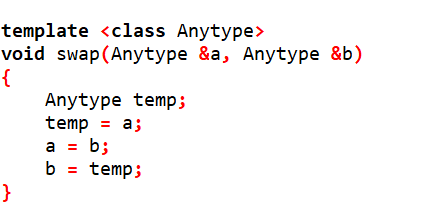调用该函数模板：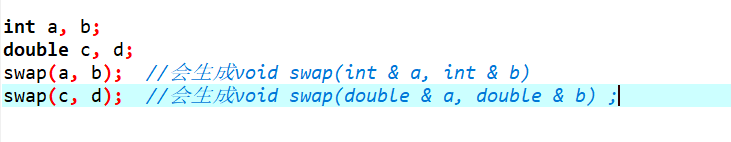重载的函数模板

例如：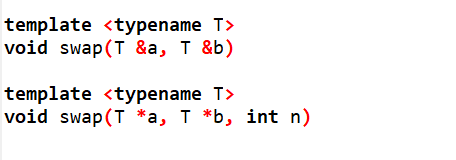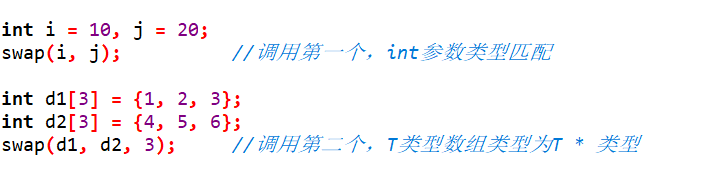模板的显示具体化

假设有一个结构体，C++允许将一个结构体赋给另一个结构体，如果只想交换其中部分成员，则需要不同的代码。

可以提供一个具体化函数定义——显示具体化。当编译器找到与函数调用匹配的具体化时，将使用该定义，而不再寻找模板。

1. 对于给定的函数名，可以有非模板函数，模板函数，和显示具体化函数以及它们的重载版本。

2.显示具体化的原型和定义应以template<>打头，并通过名称来指出类型。

3.具体化优先于常规模板，非模板函数优先于具体化和模板函数。

例如：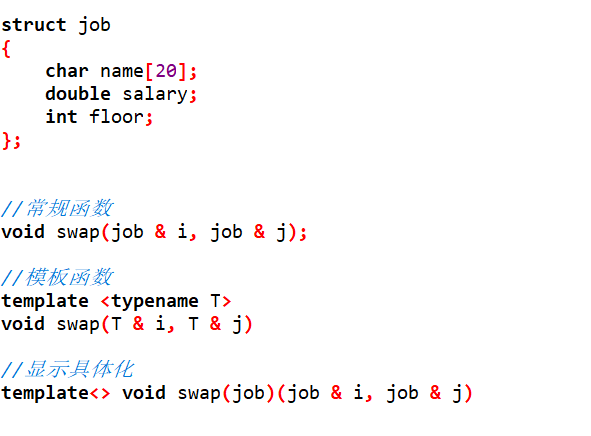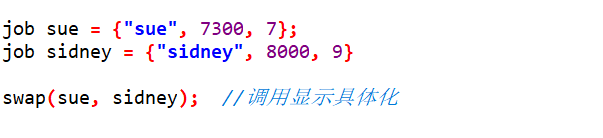实例化：

函数调用swap（i，j）导致编译器生成swap（）的一个实例，该实例使用int类型。

模板并非函数定义，但使用int的模板实例就是函数定义，这种实例化方式被称为隐式实例化。

C++允许显示实例化，template void swap<int>(int&, int & )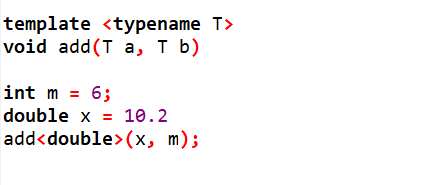编译器选择使用哪个函数版本

对于函数重载，函数模板，函数模板重载，C++有定义一个良好的策略，来决定为函数调用使用哪一个函数定义，尤其是有多个参数时，这个过程称为重载解析。

1.完全匹配，但常规函数优先于模板。

2.提升转换（char 与short自动转换为int，float自动转换为double）。

3.标准转换（int转换为char，long转换为double）。

4.用户定义的转换，如类声明中定义的转换。

函数的变量类型与返回类型

函数有多个参数类型时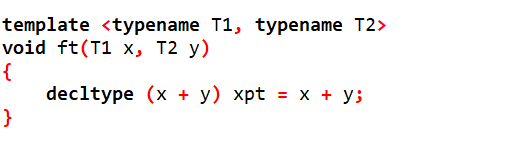x + y 的类型无法预测出来，使用decltype关键字来猜测类型。

如果函数有返回值类型：无法预先知道x + y的类型，此时还未声明参数x 和 y，他们不在作用域内（编译器无法看到它们，无法使用它们）。必须在声明参数后使用decltype。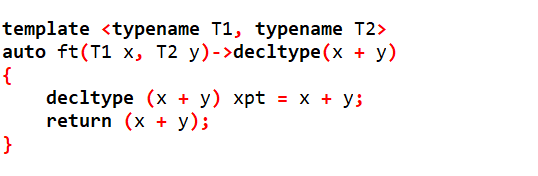现在，decltype在参数后边声明，因此x， y位于作用域内，可以使用它们。

展开全文• 谭浩强老师c++课本函数模板例题，c++函数模板使用，供大家学习使用。
• 描述C++模板类，介绍使用方式，对于初学者来说是比不可少的学习资源
• C++提供了模板(template)编程的概念。所谓模板，实际上是建立一个通用函数或类，其类内部的类型和函数的形参类型不具体指定，用一个虚拟的类型来代表。这种通用的方式称为模板模板是泛型编程的基础,泛型编程即以一...
• 为进一步简化，C++提供了函数模板，实际上是建立一个通用函数，其函数类型和形参类型不具体指定，用一个虚拟的类型来代表，此通用函数即为函数模板。在调用函数时系统会根据实参的类型来取代模板中的虚拟类型，从而...
• C++函数模板类模板 C++语言引入模板技术，它使用参数化的类型创建相应的函数和类，分别称之为函数模板和类模板 函数模板： 可以用来创建一个通用功能的函数，以支持多种不同形参，进一步简化重载函数的函数体设计 ...编程语言
• /****************类模板***/ #include <iostream> using namespace std; template <class T1,class T2> class Pair { public: T1 key;//关键字 T2 value;//值 Pair(T1 k,T2 v):key(k),v...
• 刚开始创建函数模板时,就是这种情况,当后续未调用该函数时,是不会实例化该函数的。 然后是显式实例化: template void swap<int>(int& a, int& b); /*显示实例化,无论后面是否使用了该函数,编译器都会...
• ## C++函数模板&类模板详解

千次阅读 多人点赞 2018-12-02 16:32:37
C++ 中，模板分为函数模板和类模板两种。函数模板是用于生成函数的，类模板则是用于生成类的。 函数模板&amp;模板函数 类模板&amp;模板类 必须区分概念 函数模板是模板，模板函数时具体的函数 类模板...泛型
• 模板编程中如果要特化或偏特化(局部特化)一个类模板，需要特化该类模板的所有成员函数。类模板中大多数成员函数的功能可能是一模一样的，特化时我们可能只需要重新实现1、2个成员函数即可。在这种情况下，如果全部...
• ## C++函数模板与类模板的区别

万次阅读 多人点赞 2018-07-18 16:54:55
C++ 除了支持函数模板，还支持类模板（Class Template）。函数模板中定义的类型参数可以用在函数声明和函数定义中，类模板中定义的类型参数可以用在类声明和类实现中。类模板的目的同样是将数据的类型参数化。 声明...类模板
• C++函数模板 模板实例化、具体化 函数模板是C++新增的一种性质，它允许只定义一次函数的实现，即可使用不同类型的参数来调用该函数。这样做可以减小代码的书写的复杂度，同时也便于修改（注：使用模板函数并不会减少...
• 所谓函数模板，实际上是建立一个通用函数，其函数类型和形参类型不具体指定，用一个虚拟的类型来代表。这个通用函数就称为函数模板。 凡是函数体相同的函数都可以用这个模板来代替，不必定义多个函数，只需在模板中...
• C++提供的函数模板可以更加简化这个过程。 所谓函数模板实际上是建立一个通用函数，其涵涵素类型额形参类型不具体指定，用一个虚拟的类型来代表，这个通用函数就称为函数模板。 凡是函数体相同的函数都可以用这个...
• 函数模板和普通函数区别结论： 　函数模板不允许自动类型转化 　普通函数能够进行自动类型转换 　函数模板和普通函数在一起，调用规则： 　1 函数模板可以像普通函数一样被重载 　2 C++编译器优先考虑普通函数 ...
• 利用函数模板封装一个排序的函数，可以对不同数据类型数组进行排序 排序规则从大到小，排序算法为选择排序 分别利用char数组和int数组进行测试 //交换的函数模板 template<typename T> void mySwap(T &a,...排序算法...

# c++函数模板c++ 订阅